# Sample Data: Meuse River

Annotated locations of soil samples along the Dutch bank of the Meuse River north of Maastricht

## Details

Locations of soil samples collected in a flood plain of the river Meuse, near the village of Stein (NL) in the observation region which is the convex hull of the sample locations, annotated with marks including elevation (in meters), distance to river (in meters), flooding frequency, cadmium (in ppm), copper (in ppm), lead (in ppm), zinc (in ppm), percent of organic matter, presence of lime, and soil type (Rd10A - Calcareous weakly-developed meadow soils, light sandy clay; Rd90C/VII - Non-calcareous weakly-developed meadow soils, heavy sandy clay to light clay; Bkd26/VII - Red Brick soil, fine-sandy, silty light clay).

## Examples

### Basic Examples (2)

 In:=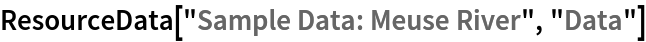Out=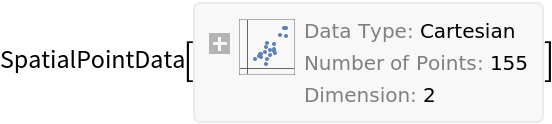Summary of the spatial point data:

 In:=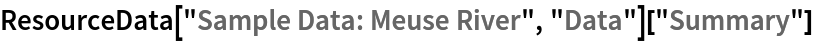Out=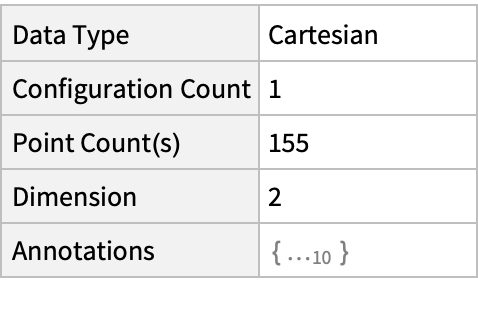List annotations keys:

 In:=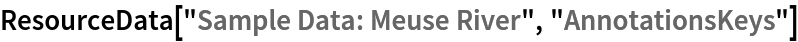Out=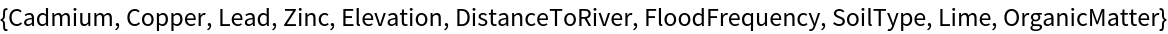### Visualizations (5)

Plot the locations:

 In:=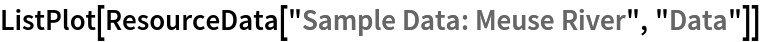Out=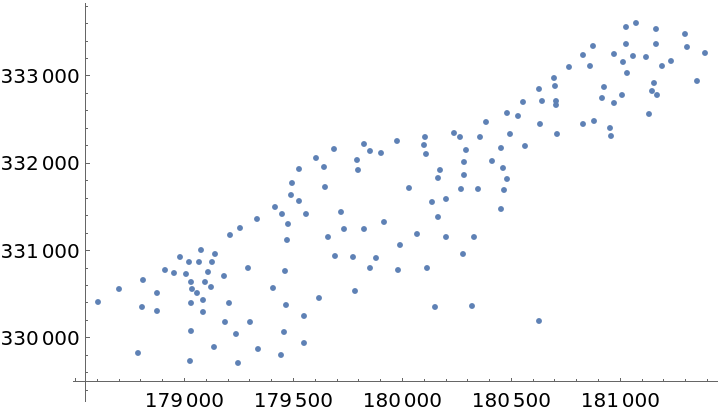Visualize the smooth point density:

 In:=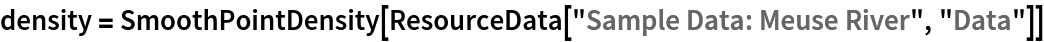Out=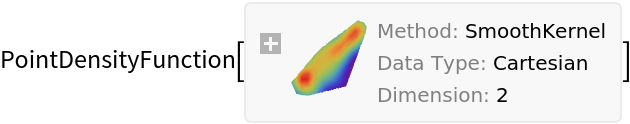In:=Out=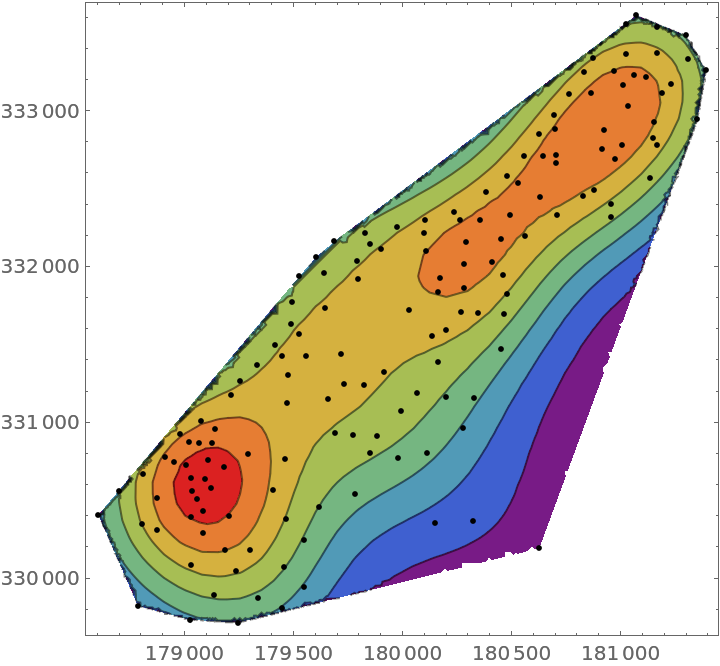Visualize points with some annotations:

 In:=Out=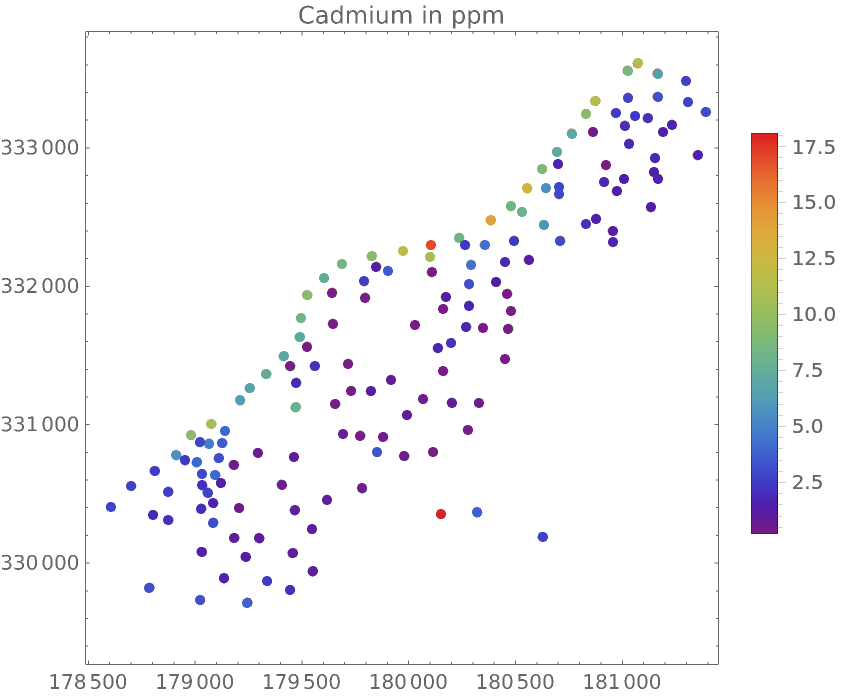Compare distributions of the four metals:

 In:=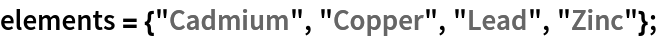In:=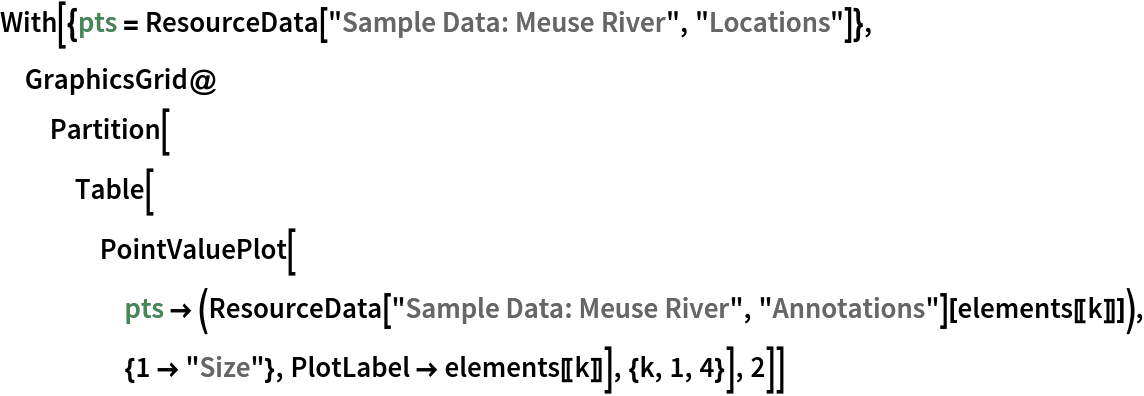Out=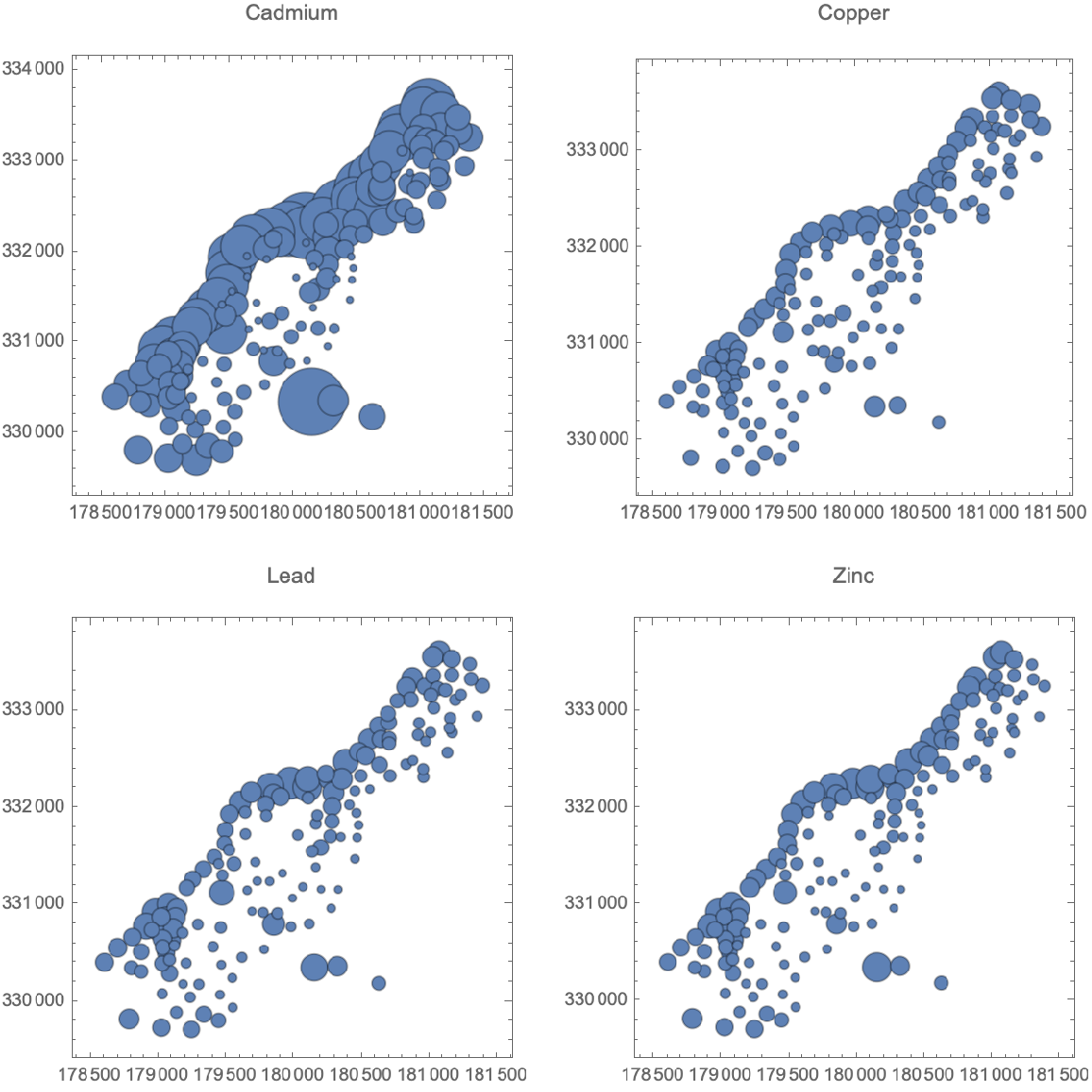Flood frequency at locations:

 In:=Out=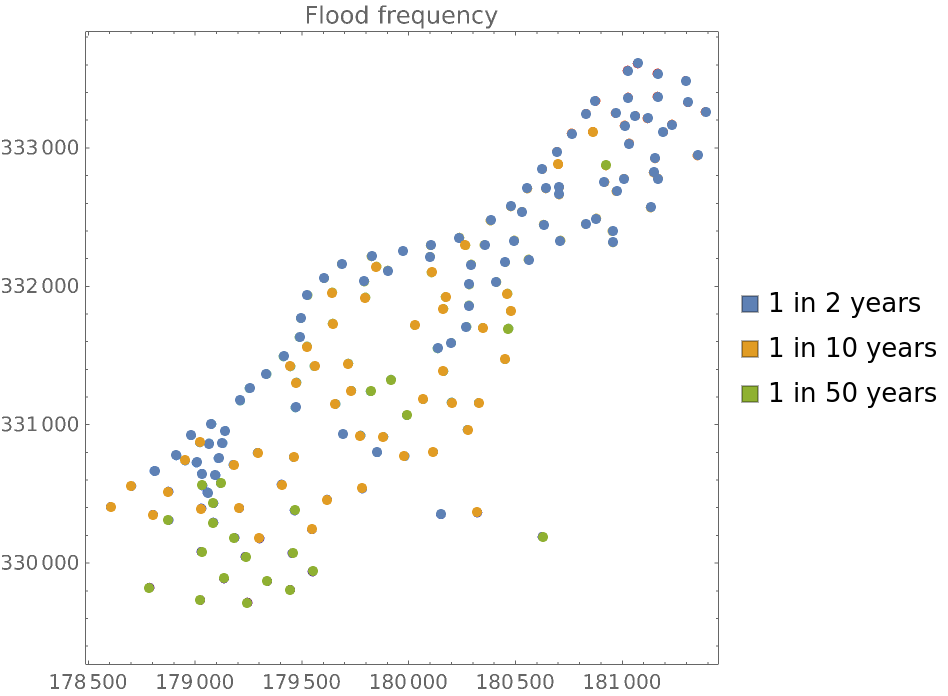### Analysis (3)

Use SpatialEstimate to create a continuous estimate from sparse observation locations:

 In:=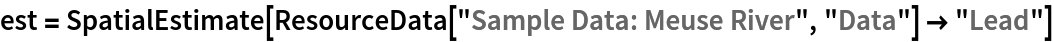Out=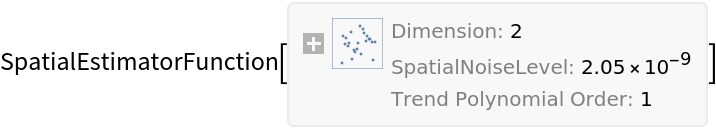Create a set of random points and compute the estimated values at these locations:
 In:=Visualize the values over the whole region:

 In:=Out=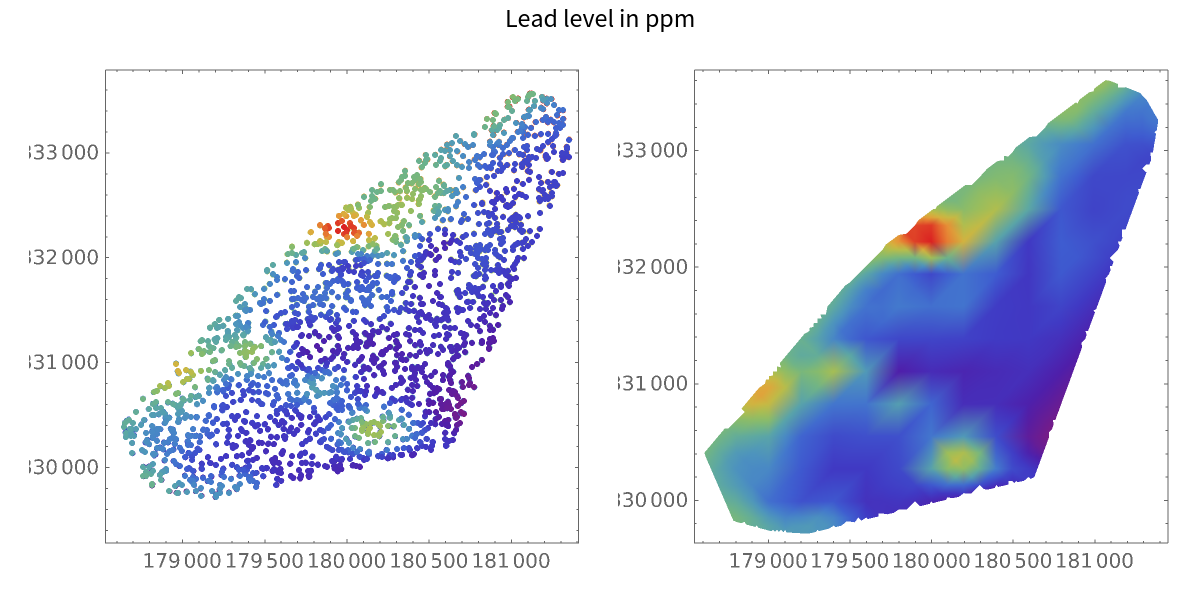Gosia Konwerska, "Sample Data: Meuse River" from the Wolfram Data Repository (2022)